# C Functions

A function in C is a set of statements that when called perform some specific task. It is the basic building block of a C program that provides modularity and code reusability. The programming statements of a function are enclosed within { } braces, having certain meanings and performing certain operations. They are also called subroutines or procedures in other languages.

In this article, we will learn about functions, function definition. declaration, arguments and parameters, return values, and many more.

## Syntax of Functions in C

The syntax of function can be divided into 3 aspects:

1. Function Declaration
2. Function Definition
3. Function Calls

## Function Declarations

In a function declaration, we must provide the function name, its return type, and the number and type of its parameters. A function declaration tells the compiler that there is a function with the given name defined somewhere else in the program.

### Syntax

`return_type name_of_the_function (parameter_1, parameter_2);`

The parameter name is not mandatory while declaring functions. We can also declare the function without using the name of the data variables.

### Example

```int sum(int a, int b);
int sum(int , int);```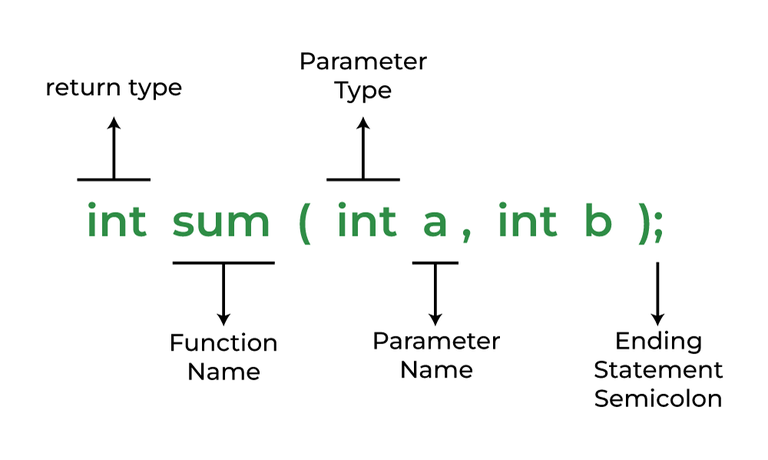Function Declaration

Note: A function in C must always be declared globally before calling it.

## Function Definition

The function definition consists of actual statements which are executed when the function is called (i.e. when the program control comes to the function).

A C function is generally defined and declared in a single step because the function definition always starts with the function declaration so we do not need to declare it explicitly. The below example serves as both a function definition and a declaration.

```return_type function_name (para1_type para1_name, para2_type para2_name)
{
// body of the function
}```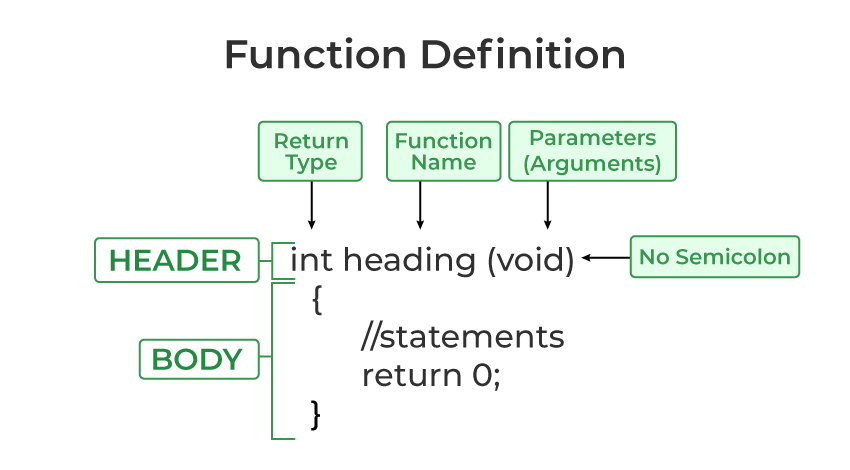Function Definition in C

## Function Call

A function call is a statement that instructs the compiler to execute the function. We use the function name and parameters in the function call.

In the below example, the first sum function is called and 10,30 are passed to the sum function. After the function call sum of a and b is returned and control is also returned back to the main function of the program.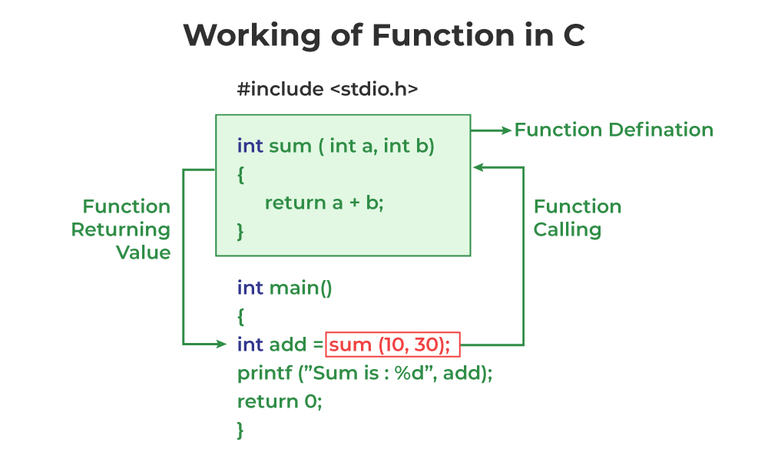Working of function in C

Note: Function call is neccessary to bring the program control to the function definition. If not called, the function statements will not be executed.

## C

 `// C program to show function` `// call and definition` `#include `   `// Function that takes two parameters ` `// a and b as inputs and returns ` `// their sum` `int` `sum(``int` `a, ``int` `b) ` `{ ` `  ``return` `a + b; ` `}`   `// Driver code` `int` `main()` `{` `  ``// Calling sum function and ` `  ``// storing its value in add variable` `  ``int` `add = sum(10, 30);` `  `  `  ``printf``(``"Sum is: %d"``, add);` `  ``return` `0;` `}`

Output

`Sum is: 40`

As we noticed, we have not used explicit function declaration. We simply defined and called the function.

### Function Return Type

Function return type tells what type of value is returned after all function is executed. When we don’t want to return a value, we can use the void data type.

Example:

`int func(parameter_1,parameter_2);`

The above function will return an integer value after running statements inside the function.

Note: Only one value can be returned from a C function. To return multiple values, we have to use pointers or structures.

### Function Arguments

Function Arguments (also known as Function Parameters) are the data that is passed to a function.

Example:

`int function_name(int var1, int var2);`

## Conditions of Return Types and Arguments

In C programming language, functions can be called either with or without arguments and might return values. They may or might not return values to the calling functions.

1. Function with no arguments and no return value
2. Function with no arguments and with return value
3. Function with argument and with no return value
4. Function with arguments and with return value

To know more about function Arguments and Return values refer to the article – Function Arguments & Return Values in C.

## How Does C Function Work?

Working of the C function can be broken into the following steps as mentioned below:

1. Declaring a function: Declaring a function is a step where we declare a function. Here we define the return types and parameters of the function.
2. Defining a function:
3. Calling the function: Calling the function is a step where we call the function by passing the arguments in the function.
4. Executing the function: Executing the function is a step where we can run all the statements inside the function to get the final result.
5. Returning a value: Returning a value is the step where the calculated value after the execution of the function is returned. Exiting the function is the final step where all the allocated memory to the variables, functions, etc is destroyed before giving full control to the main function.

## Types of Functions

There are two types of functions in C:

1. Library Functions
2. User Defined Functions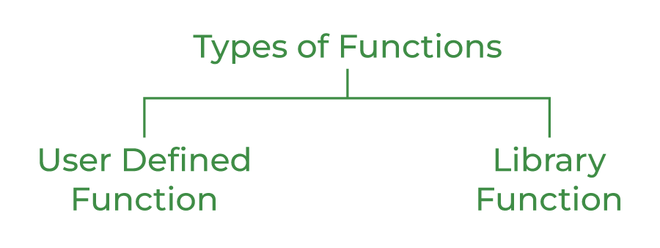Types of Functions in C

### 1. Library Function

A library function is also referred to as a “built-in function”. A compiler package already exists that contains these functions, each of which has a specific meaning and is included in the package. Built-in functions have the advantage of being directly usable without being defined, whereas user-defined functions must be declared and defined before being used.

For Example:

`pow(), sqrt(), strcmp(), strcpy() etc.`

#### Advantages of C library functions

• C Library functions are easy to use and optimized for better performance.
• C library functions save a lot of time i.e, function development time.
• C library functions are convenient as they always work.

Example:

## C

 `// C program to implement` `// the above approach` `#include ` `#include `   `// Driver code` `int` `main()` `{` `  ``double` `Number;` `  ``Number = 49;`   `  ``// Computing the square root with ` `  ``// the help of predefined C ` `  ``// library function` `  ``double` `squareRoot = ``sqrt``(Number);` `  `  `  ``printf``(``"The Square root of %.2lf = %.2lf"``, ` `          ``Number, squareRoot);` `  ``return` `0;` `}`

Output

`The Square root of 49.00 = 7.00`

### 2. User Defined Function

Functions that the programmer creates are known as User-Defined functions or “tailor-made functions”. User-defined functions can be improved and modified according to the need of the programmer. Whenever we write a function that is case-specific and is not defined in any header file, we need to declare and define our own functions according to the syntax.

• Changeable functions can be modified as per need.
• The Code of these functions is reusable in other programs.
• These functions are easy to understand, debug and maintain.

Example:

## C

 `// C program to show ` `// user-defined functions` `#include `   `int` `sum(``int` `a, ``int` `b) ` `{ ` `  ``return` `a + b; ` `}`   `// Driver code` `int` `main()` `{` `  ``int` `a = 30, b = 40;` ` `  `  ``// function call` `  ``int` `res = sum(a, b);`   `  ``printf``(``"Sum is: %d"``, res);` `  ``return` `0;` `}`

Output

`Sum is: 70`

## Passing Parameters to Functions

The data passed when the function is being invoked is known as the Actual parameters. In the below program, 10 and 30 are known as actual parameters. Formal Parameters are the variable and the data type as mentioned in the function declaration. In the below program, a and b are known as formal parameters.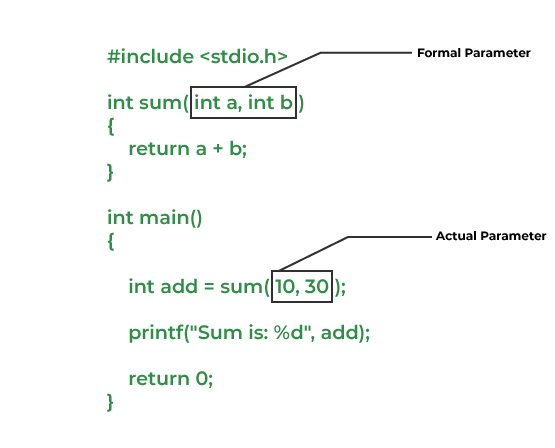Passing Parameters to Functions

We can pass arguments to the C function in two ways:

1. Pass by Value
2. Pass by Reference

### 1. Pass by Value

Parameter passing in this method copies values from actual parameters into formal function parameters. As a result, any changes made inside the functions do not reflect in the caller’s parameters.

Example:

## C

 `// C program to show use ` `// of call by value` `#include `   `void` `swap(``int` `var1, ``int` `var2)` `{` `  ``int` `temp = var1;` `  ``var1 = var2;` `  ``var2 = temp;` `}`   `// Driver code` `int` `main()` `{` `  ``int` `var1 = 3, var2 = 2;` `  ``printf``(``"Before swap Value of var1 and var2 is: %d, %d\n"``,` `          ``var1, var2);` `  ``swap(var1, var2);` `  ``printf``(``"After swap Value of var1 and var2 is: %d, %d"``,` `          ``var1, var2);` `  ``return` `0;` `}`

Output

```Before swap Value of var1 and var2 is: 3, 2
After swap Value of var1 and var2 is: 3, 2```

### 2. Pass by Reference

The caller’s actual parameters and the function’s actual parameters refer to the same locations, so any changes made inside the function are reflected in the caller’s actual parameters.

Example:

## C

 `// C program to show use of ` `// call by Reference` `#include `   `void` `swap(``int` `*var1, ``int` `*var2)` `{` `  ``int` `temp = *var1;` `  ``*var1 = *var2;` `  ``*var2 = temp;` `}`   `// Driver code` `int` `main()` `{` `  ``int` `var1 = 3, var2 = 2;` `  ``printf``(``"Before swap Value of var1 and var2 is: %d, %d\n"``,` `          ``var1, var2);` `  ``swap(&var1, &var2);` `  ``printf``(``"After swap Value of var1 and var2 is: %d, %d"``,` `          ``var1, var2);` `  ``return` `0;` `}`

Output

```Before swap Value of var1 and var2 is: 3, 2
After swap Value of var1 and var2 is: 2, 3```

## Advantages of Functions in C

Functions in C is a highly useful feature of C with many advantages as mentioned below:

1. The function can reduce the repetition of the same statements in the program.
2. The function makes code readable by providing modularity to our program.
3. There is no fixed number of calling functions it can be called as many times as you want.
4. The function reduces the size of the program.
5. Once the function is declared you can just use it without thinking about the internal working of the function.

## Disadvantages of Functions in C

The following are the major disadvantages of functions in C:

1. Cannot return multiple values.
2. Memory and time overhead due to stack frame allocation and transfer of program control.

## Conclusion

1. The function is the block of code that can be reused as many times as we want inside a program.
2. To use a function we need to call a function.
3. Function declaration includes function_name, return type, and parameters.
4. Function definition includes the body of the function.
5. The function is of two types user-defined function and library function.
6. In function, we can according to two types call by value and call by reference according to the values passed.

## FAQs on Functions in C

### Q1. Define functions.

Functions are the block of code that is executed every time they are called during an execution of a program.

### Q2. What is a forward declaration?

Sometimes we define the function after its call to provide better readibliy. In such cases, we declare function before the their defiinition and call. Such declaration are called Forward Declaration.

### Q3. What is the difference between function declaration and definition?

The data like function name, return type, and the parameter is included in the function declaration whereas the definition is the body of the function. All these data are shared with the compiler according to their corresponding steps.

### Q4. What is the difference between function arguments and parameters?

Function parameters are the values declared in a function declaration. Whereas, function arguments are the values that are passed in the function during the function call.

Example:

```int func(int x,int y);
func(10,20);```

Here, int x and int y are parameters while, 10 and 20 are the arguments passed to the function.

To know more about it, refer to this article – Difference between Arguments and Parameters in C.

### Q5. Can we return multiple values from a C Function?

No, it is generally not possible to return multiple values from a function. But we can either use pointers to static or heap memory locations to return multiple values or we can put data in the structure and then return the structure.

To know more about it, refer to this article – How to return multiple values from a function in C or C++?